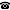# 2019 seminar talk: Ramsey Theory of the Henson graphs

Talk held by Natasha Dobrinen (University of Denver, Colorado, USA) at the KGRC seminar on 2019-01-10.

### Abstract

A central question in the theory of ultrahomogeneous relational structures asks, How close of an analogue to the Infinite Ramsey Theorem does it carry? An infinite structure $\mathbf{S}$ is ultrahomogeneous if any isomorphism between two finitely generated substructures of $\mathbf{S}$ can be extended to an automorphism of $\mathbf{S}$. We say that $\mathbf{S}$ has finite big Ramsey degrees if for each finite substructure $A$ of $\mathbf{S}$, there is a number $n(A)$ such that any coloring of the copies of $A$ in $\mathbf{S}$ can be reduced to no more than $n(A)$ colors on some substructure $\mathbf{S}'$ of $\mathbf{S}$, which is isomorphic to the original $\mathbf{S}$.

The two main obstacles to a fuller development of this area have been lack of representations and general Milliken-style theorems. We will present new work proving that the Henson graphs, the $k$-clique free analogues of the Rado graph for $k\ge 3$, have finite big Ramsey degrees. We devise representations of Henson graphs via strong coding trees and prove Millike-style theorems for these trees. Central to the proof is the method of forcing, building on Harrington's proof of the Halpern-Läuchli Theorem.

There is a video recording of this talk on YouTube.

Slides are available, too.

Kurt Gödel Research Center for Mathematical Logic. Währinger Straße 25, 1090 Wien, Austria.+43-1-4277-50501. Last updated: 2010-12-16, 04:37.